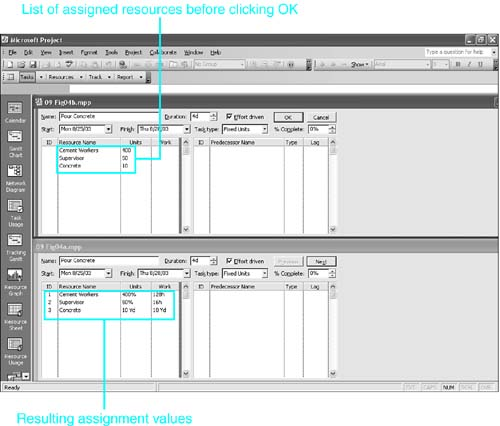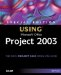# Understanding the Work Formula

 <  Day Day Up  >

As described previously in this chapter, Project uses assignment units as a multiplier to calculate how much work it must schedule. When you assign two carpenters (200%) to work on a task with a duration of 4 hours, Project schedules 2 hours of work for each of the 4 hours of duration. The total work is therefore 8 hours.

The formula for calculating work is as follows :

Duration x Units = Work

In symbols, it is represented as follows:

D x U = W

That is, you multiply the task duration by the assigned units to calculate work.

NOTE

These formulas do not apply to assignments for material resources that have a fixed consumption rate. For those assignments, the value in the Work field is identical to the value in the Units field. The formulas do apply to material resources that have variable consumption rates. However, these formulas were developed and implemented before the material resource type was introduced, and the following discussion makes more sense if you think in terms of work resources.

Simple algebra can be used to reformulate this equation to calculate values for Duration when Work and Units are given:

Duration = Work / Units

In symbols, it looks like this:

D = W / U

Also, when Duration and Work are given, Project can calculate Units with this variation of the formula:

Units = Work / Duration

In symbols, it looks like this:

U = W / D

Although the duration can be displayed in minutes, hours, days, weeks, or months, Project converts the duration to minutes when calculating work and then displays it in the default unit for work, which is normally the hour . Thus, if a 1-day task has 100% units assigned to it (that is, one full-time unit of the resource), the work is calculated as follows:

D x U = W

8 hrs x 100% = 8 hrs

#### Applying the Work Formula in New Assignments

To ingrain your understanding of how Project calculates the changes brought about by assigning resources, we will examine the simplest case first ”where you assign a single resource in an initial assignment. Then we will examine the case where you assign multiple resources in an initial assignment. After that, we will examine how Project responds when you change existing assignments.

You should experiment with each of these cases to solidify your understanding. Display the Gantt Chart view and use the Window, Split command to display the Task Form view in the bottom pane. Right-click over the Task Form view and choose Resources and Predecessors from the Detail list. The Assignment Units and Work fields appear next to the resource name in the resource details (refer to Figure 9.2). Try different values for units and work until you feel you can predict the outcome when you create an assignment.

##### Assigning a Single Resource in an Initial Assignment

The duration for a task is already defined before you assign the first resource to the task. Therefore, one of the values in the work formula ”task duration ”is already established. Project handles your entries when assigning the initial resource slightly differently for work and material resources, so we will consider them separately.

##### Entering a New Assignment for Work Resources

The following cases show what happens for the initial assignment of a work resource. The distinctions are based on which fields you fill in: Units only, Work only, or both Units and Work. All the cases assume that the task has a duration of 1 day, and that days and weeks are defined to be 8 hours and 40 hours, respectively. They also assume that the task type is Fixed Units (see "Choosing the Task Type," later in this chapter, for more information).

Project's responses to your entries when creating a work resource assignment are as follows:

• If you enter the resource name when creating the assignment and you don't provide the values for units or work, Project assumes that you want the default units (usually 100%) and calculates the work from the duration and units. For example, if you assign a work resource to a 1-day task without specifying the units, Project supplies 100% to the Units field and calculates 8 hours for the Work field, as follows:

D x U = W

8 hrs x 100% = 8 hrs

• If you enter the Units value, Project uses that value with the duration to calculate the work. For example, if you assign a resource to the 1-day task and enter 50% in the Units field, Project calculates the work as follows:

D x U = W

8 hrs x 50% = 4 hrs

The task duration is still 1 day.

• If you enter the Work value but do not supply the units, Project assumes that you want the default units (usually 100%) and calculates a new value for the duration, based on the specified work and the assumed value for units. For example, if you assign a resource to the 1-day task and enter 16 hours in the Work field but leave the Units field blank, Project calculates a new Duration value as follows, because it takes more than 1 day for a single resource unit to complete 16 hours of work:

D x U = W

D x 100% = 16h

D = 16h / 100% = 16 hours (that is, 2 days)

• If you enter both the Units and Work values, Project recalculates the existing task duration, using the new entries. For example, if you assign Units the value 200% and Work the value 32 hours, Project calculates a new task Duration by using this variation of the work formula:

D x U = W

D x 200% = 32h

D = 32h / 200% = 16 hours (that is, 2 days)

##### Entering a New Assignment for Material Resources

When the resource is a material resource, there are fewer alternatives to consider than with work resources. Again, assume that the task is a 1-day task. This time, the resource is the material resource Concrete, and its label is Yd. Recall that for material resources, the Units field is always displayed in decimal format. Project's responses to your entries when creating a material resource assignment are as follows:

• If you just enter the material resource name but don't provide either the Units value or the Work value of the assignment, Project assumes that the Units value is 1 and calculates the work to be 1 Yd. For example, if you assign the Concrete resource to a 1-day task without specifying the units, Project supplies 1 Yd to both the Units and Work fields.

• If you enter the Units value as a numeral (in other words, as a fixed consumption rate), Project enters that value into both the Units and the Work fields. For example, if you assign the Concrete resource to the 1-day task and enter 2 in the Units field, Project supplies 2 Yd to both the Units and Work fields.

• If you enter the units value as a variable consumption rate, Project uses that rate with the task duration to calculate the Work field. For example, if you enter 2/d in the Units field, Project converts that to 2 Yd/day and multiplies that value by the duration (1 day) to calculate the Work field entry 2 Yd.

• If you enter the Work value but do not supply the units, Project assumes that the amount you entered has a fixed consumption rate and puts that amount in both the Units and Work fields.

• If you enter a number in the Units field (a fixed consumption rate) and also enter a number in the Work field, Project ignores the entry in the Units field and places the Work field entry in both the Units and Work fields.

• If you enter a variable consumption rate in the Units field and also enter a number in the Work field, Project recalculates the duration so that the consumption rate yields the total units in the Work field.

##### Using Multiple Resources in an Initial Assignment

One of the advantages of using the Task Form view to create assignments is that the initial assignment can include all resources that are to be assigned to the task, and you can specify units and/or work for each assignment. Other methods of assigning resources require you to complete each assignment individually or don't let you regulate both units and work. As you will see in later sections of this chapter, individually assigning multiple resources to a task creates additional variations in how Project calculates the task work and duration. Therefore, listing all the resources in the initial assignment sometimes saves you time and annoying hassles.

CAUTION

You must be very careful when including variable-consumption-rate material resources along with work resources in an initial assignment. Project often calculates unexpected results for this combination. In fact, you should include in the initial assignment only the work resources and any fixed-consumption-rate material resources; you can add the variable-consumption-rate material resources later.

To create multiple assignments with the initial assignment, you simply enter multiple rows in the resource details table before you click the OK button. Project then calculates each of the assignments individually, using the principles outlined previously and using the existing task duration in each calculation.

For example, Figure 9.4 shows two task forms. The top form shows the initial assignment being created, and the bottom form shows the result after the OK button is clicked. The task is a 4-day task; four cement workers are being assigned, along with a supervisor who will spend only 50% of her time on the task. The task will also consume 10 cubic yards of concrete.

##### Figure 9.4. You can initially assign multiple resources by listing them all before clicking OK.#### Applying the Work Formula to Changes in Existing Assignments

In the examples so far in this chapter, it has seemed that Project is free to recalculate any of the three variables in the work formula. However, in the next section you will see that one of the three variables in the equation is always fixed for a task, and therefore it is fixed for any assignments to that task. Project is not allowed to change the fixed value when it has to recalculate the work equation for an assignment.

For example, by default, tasks are created as Fixed Units tasks , as indicated by the underline in the following formula:

D x U = W

Thus, if you change the duration, Project has to leave the units unchanged and must recalculate the work, or if you change the work, Project has to change the duration. You can define which variable is fixed for a task and is therefore fixed for all that task's assignment calculations. In this way you can control how Project responds when you make changes in assignments.

 <  Day Day Up  >Special Edition Using Microsoft Office Project 2003
ISBN: 0789730723
EAN: 2147483647
Year: 2004
Pages: 283
Authors: Tim Pyron

Similar book on Amazon